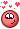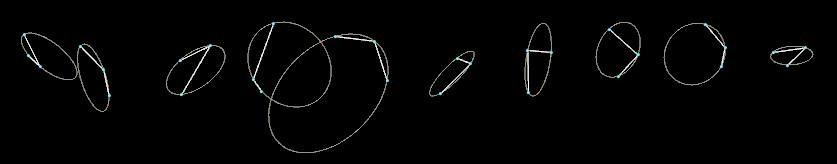## Recommended Posts

Please, do you have a vex code for creating Arc from 3 points?
From time to time it would be useful to have it.

AndrewVK shared this C++ source here:
https://www.sidefx.com/forum/topic/19104/?page=1#post-89849

I could rewrite it as an exercise and then I will share it ... but if anybody has it done, it would be a great time saver.

##### Share on other sites
Posted (edited)

HeavenMaybe it helps!

Edited by Librarian
•1

##### Share on other sites

Tesan, thank you very much for your hip file! Please, do you anybody have also a solution for a circular arc?

Here is a screenshot from the file, for a future reference:

And the complete wrangle is:

```// Convert the umap range from 0-1 to 0-1-0
float curveToHalf(
float value;
) {

return clamp(value, 0, 0.5) * clamp(fit(value, 0, 1, 1, 0) , 0, 0.5) * 4;
}
// Complement function
float complement(
float value;
) {

return 1 + (value * -1);
}
// Main interpolation function
float interpolate_curve(
float value;
string sign;
) {

float pi_half = 1.57079632679;

value = cos(value * pi_half);

if ( sign == "-" )
return 1 + (value * -1);
else
return value;

}
// Get user parameters from the UI
string  sign            = ch("sign");
int             complement      = int(ch("complement"));
int             curve_half      = int(ch("curve_half"));

// Create our classic umap attribute ranging over the points from 0-1
float   umap = float(@ptnum) / (npoints(@OpInput1) - 1);

// Convert the umap range
if ( curve_half == 1)
umap = curveToHalf(umap);

// Do the complement for the umap
if ( complement == 1)
umap = complement(umap);

// Interpolate curve based on the parameters
@P.y = interpolate_curve(umap, sign);```

##### Share on other sites

I have 100 % somewhere more files ..just need to find..and those  vex codes don't know Owner•1

##### Share on other sites

Hi,

I've found an older file. Here is a modification of it.

three_point_arc.hipnc

•1
•1

##### Share on other sites

Hi Jiri,

to get started I just wrote a script that turns a bunch of lines with three points into circles:It's basically calculating the intersection point and radius of rectangular vectors starting from the lines' midpoints. To be run in a primitive wrangle:

```// 3D INTERSECTION
// https://stackoverflow.com/questions/10551555/need-an-algorithm-for-3d-vectors-intersection
function vector intersection(vector r1, r2, e1, e2){
float u = dot(e1, e2);
float t1 = dot(r2 - r1, e1);
float t2 = dot(r2 - r1, e2);
float d1 = (t1 - u * t2) / (1 - u * u);
float d2 = (t2 - u * t1) / (u * u - 1);
vector p1 = r1 + e1 * d1;
vector p2 = r2 + e2 * d2;
vector pos_center = (p1 + p2) * 0.5;
return pos_center;
}

// INPUT POSITIONS
int pt_0 = primpoint(0, i@primnum, 0);
int pt_1 = primpoint(0, i@primnum, 1);
int pt_2 = primpoint(0, i@primnum, 2);

vector pos_A = point(0, 'P', pt_0);
vector pos_B = point(0, 'P', pt_1);
vector pos_C = point(0, 'P', pt_2);

vector mid_AB = (pos_A + pos_B) * 0.5;
vector mid_BC = (pos_B + pos_C) * 0.5;

// DIRECTIONS
vector dir_BA = normalize(pos_B - pos_A);
vector dir_BC = normalize(pos_B - pos_C);
vector dir_rect = normalize(cross(dir_BA, dir_BC));
vector dir_BA_rect = normalize(cross(dir_BA, dir_rect));
vector dir_BC_rect = normalize(cross(dir_BC, dir_rect));

vector pos_center = intersection(mid_AB, mid_BC, dir_BA_rect, dir_BC_rect);
matrix3 xform_circle = dihedral({0,0,1}, dir_rect);

// REMOVE INPUT GEOMETRY
removeprim(0, i@primnum, 1);```

circles_from_points.hipnc

•2
•2

## Create an account

Register a new account